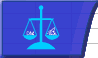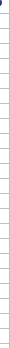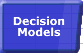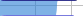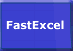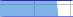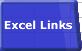# Excel DependenciesSmart Recalculation Evaluation Circumstances Calculation Process Dependency Trees Controlling Calculation "CALCULATE" in Status Bar Calculation Methods Calculating from VBA Volatile Functions & Actions User-Defined Functions Repetitive Calculation Version Timing Comparison## Dependency Trees

 Dependencies are important to fast recalculation. Excel tracks what has changed since the last recalculation, and builds Dependency Trees to attempt to minimise calculation time. These allow Excel to recalculate only: Formulae/Names that have changed. Formulae containing Volatile Functions Formulae dependent on changed or volatile formulae or cells or names. Excel determines dependencies by looking at the cells referred to by each formula and by the argument list of each function. Dependency trees are immediately updated whenever a formula is entered or changed. In Excel 2002 and later you can force the dependency trees to be rebuilt and all formulae calculated using CTL/ALT/SHIFT/F9

In a complex workbook Excel may spend significant time and use large amounts of memory building and evaluating the dependency trees, and will only track a maximum of 65,536 dependencies to unique references for version prior to Excel 2007. When that limit is reached Excel no longer requires to use the memory needed for the dependency trees. If you have a workbook which is just short of that limit you may find that a Full Calculation is faster than a Recalculation. In Excel 2007 these limits on the size of the dependency tree have been removed.

Experiments indicate that dependencies are handled as follows in Excel97, Excel2000, Excel 2002, Excel 2003 and Excel 2007:

### Within Sheet Dependency Trees:

 Within sheet dependency trees are dynamically maintained each time a worksheet is altered. Excel builds a Dependency Tree for each changed cell/name on each worksheet using something like the following algorithm: Whenever a cell/name is changed: Flag the changed cell/name as uncalculated. Scan the worksheet top-to-bottom and left-to-right looking for cells that refer to an uncalculated cell/name, and flag them as uncalculated. Repeat the scan as many times as necessary to resolve all dependencies. In extreme forward referencing cases with a deep dependency tree (a long chain of formulae) this process can take a significant amount of time: if changing a cell and tabbing to the next cell takes a long time you may have hit this problem. In Excel 2007 you can switch off the dependency tree rebuild using Activeworkbook.ForceFullCalculation=True. The dependency tree is updated at each change even when calculation is set to manual. Within-sheet forward dependencies do not usually have any significant effect on calculation/recalculation time.

### Inter Sheet Dependency Trees:

 Inter Sheet Dependencies can increase calculation times significantly. Excel 2002 handles inter sheet dependencies significantly better than Excel 97 and 2000. Calculating a reference to a cell on the same worksheet is faster than calculating a reference to a cell on another worksheet. Excel 97 and 2000 maintain a sheet-level dependency tree rather than a full dependency tree at the cell level for worksheet cross-references. Worksheets will be correctly flagged as containing uncalculated cells when they contain formulae dependent on an uncalculated cell/name on another worksheet. Excel 97 and 2000 handle inter-sheet dependencies by calculating each sheet containing uncalculated cells in turn, in the sequence given by their alphabetic names. The calculation process for each sheet only looks at cells that are currently flagged as uncalculated. If each sheet only refers backwards then the process is complete after each sheet has been calculated once, and all cells and sheets will have been flagged as calculated. If any sheets contain cells with uncalculated Forward Worksheet Cross-references, (references to uncalculated formulae on worksheets that are calculated after the current worksheet), these cells cannot yet be calculated and so the worksheet will still be flagged as containing uncalculated cells. This process continues until there are no remaining uncalculated sheets and cells. Forward Worksheet Cross-references (forward inter Sheet dependencies) can cause uncalculated cells to be evaluated multiple times and can increase your calculation times significantly. When these forward worksheet cross-references become linked together into one or more circular worksheet cross-reference paths calculation time can increase even more. You can detect this effect with FastExcel by looking at the Workbook Overhead: this shows the amount of time due to forward worksheet cross-references. You can also analyse the worksheet cross-references by using the FastExcel Map CrossReferences command. This will give you a recommended worksheet calculation sequence, and show you the circular worksheet cross-reference paths it has found. Excel 2002/2003 has a significantly improved calculation algorithm for handling forward worksheet cross-references. Excel2002/2003 also calculates worksheets in alphabetic sequence but develops a single dependency chain sequence for each workbook. For complex workbooks containing many forward worksheet references and/or circular worksheet references using Excel 2002/2003 can significantly speed up calculation times.

### Inter Workbook Dependencies

These are handled similarly to inter sheet dependencies, and therefore complex forward inter workbook dependencies will increase your calculation times significantly.

### Names

Names are only calculated when they are referred to, directly or indirectly, by formulae on the worksheet: unused names are not calculated even by full Calculation (Ctrl_Alt-F9).© 2001-2020 Decision Models Legal Disclaimer Privacy Statement